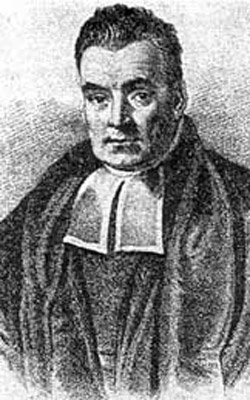A# Bayes, Thomas (1702–1761)Thomas Bayes was an English mathematician and theologian, remembered chiefly for the theorem named after him and the technique of Bayesian inference that arises from it. He also wrote more generally on probability theory, the logical basis of calculus, and asymptotic series. Bayes' proved a special case of what's now known as Bayes' theorem in An Essay towards solving a Problem in the Doctrine of Chances (1763).

## Bayes' theorem

Bayes' theorem, also known as Bayes' rule, is used in statistical inference to update estimates of the probability that different hypotheses are true, based on observations and a knowledge of how likely those observations are, given each hypothesis. In fact, it is habitually used by scientists in preference to the principle of induction.

For example, let's say a man wakes up in the morning and the moment after the sun rises he hears a rooster crow. If this happens once or twice he might simply take note of it, but if it happens repeatedly for 25 or 35 days, or even months or years, it is highly likely that he will form a link between the events: the probability of the rooster crowing the moment after the sun rises is true (it is very likely to carry on happening in the future).

The formula looks like this : Pr(A|B) = Pr(B|A) × Pr(A) / Pr(B). On the left side of the equation is the conditional probability, or what we want to know – the probability of event A (the rooster crowing) if event B (the sun rising) happens or is true. The part of the formula on the right side of the equation gives us the tools for finding the conditional probability. It involves assigning numerical values to the three components; basically, it gives numerical weight to how commonly or often A occurs, B occurs, and A and B occur together. The higher the numbers are for A, for B, and for A and B together, the more probable or likely A occurs, given B.

Bayes' theorem is important because constantly trying to calculate conditional probabilities is a natural response on the part of humankind to existing in a largely ungovernable material world. People wake up with a very strong expectation that daylight will appear, that gravity is still in place, that their cars will start, and other similar inductive inferences, and they form these beliefs because of consistent, repeated occurrences and relationships.

## Bayesian inference

Bayesian inference is a form of statistical inference in which probabilities are interpreted not as frequencies or proportions, but rather as degrees of belief. A prior distribution for a certain random variable is assumed; then this is modified, in the light of experimentation, using Bayes' theorem. Pierre Laplace applied Bayesian inference to estimate the mass of Saturn and in a variety of other problems.